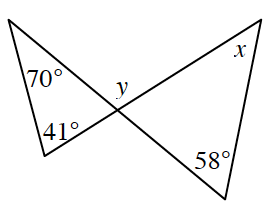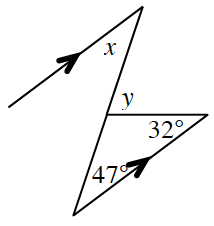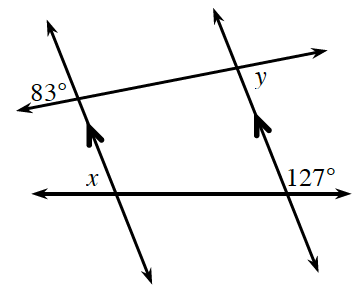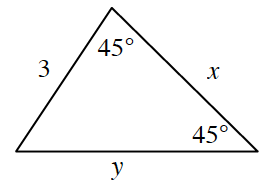### Home > INT2 > Chapter 6 > Lesson 6.1.3 > Problem6-33

6-33.

Use the relationships found in each of the diagrams below to solve for $x$ and $y$. Assume the diagrams are not drawn to scale. State which geometric relationships you used.

1.What is the measure of the missing angle in the left triangle? Once you know that angle, calculate the measure of angle $y$.

$y = 111^\circ$; $x = 53^\circ$
Triangle Angle Sum Theorem and ____

1.Parallel lines create alternate interior angles. Which angles are alternate interior angles in this diagram?

1.Redraw the diagram with only the parallel lines and the line at the top. Then determine the value of $y$.

1.This diagram is not drawn to scale. Determine the measure of the missing angle then draw the diagram to scale. What special triangle is this?